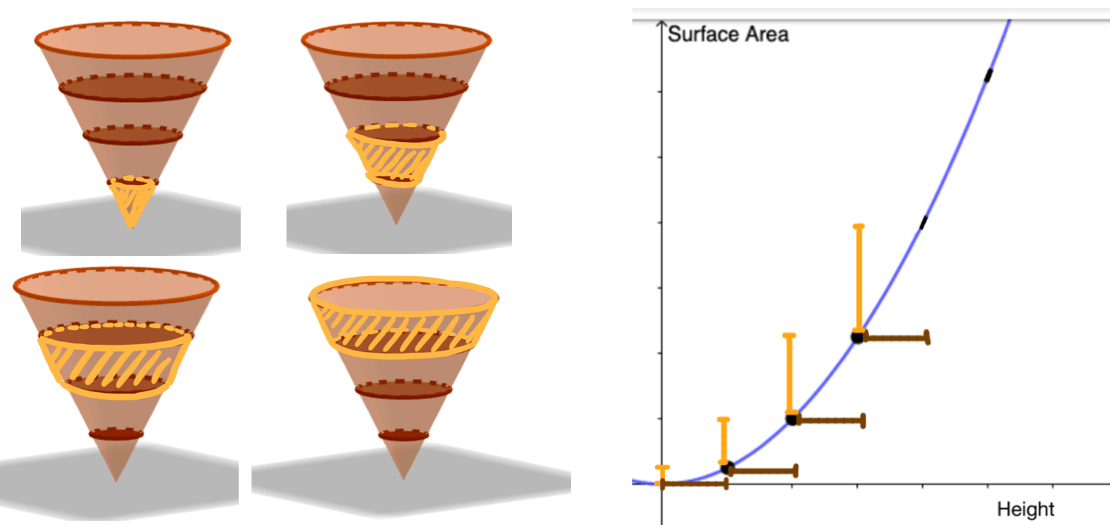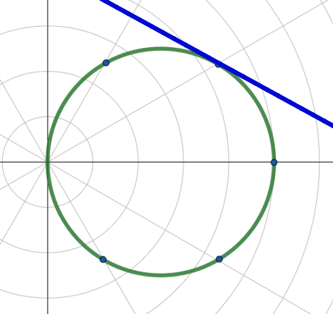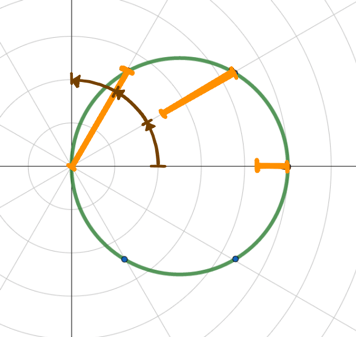# Understanding in Calculus: Beyond the “Sliding Tangent Line”

By: Natalie Hobson, Sonoma State University

If you give calculus students graphs, they are going to draw tangent lines. As instructors we often encourage students to rely on tangent lines so heavily that discussions about rates of change become lessons about sliding lines along graphs, rather than about understanding the relationships that these graphs represent in the context of a given problem.

So how do we help students develop a deeper understanding of these relationships? Let’s consider an exercise that you might give your own students during a lesson on tangent lines and rates of change.

Exercise 1: The Growing Cone

The images in Figure 1 (left) depict a growing cone. The graph in Figure 1 (right) represents the relationship between the outer surface area and height of the growing cone. Describe the rate of change of the surface area with respect to the height of the cone as it grows.Figure 1: The growing cone exercise. Image of a growing cone (left) and corresponding graph (right).

## A Common Response: The “Sliding Tangent Line”

A typical solution to the Growing Cone exercise involves drawing a collection of tangent lines along the graph in Figure 1 and exploring the steepness of these lines as the height increases (see Figure 2). One could observe that the tangent lines become steeper, thus the rate of change increases.Figure 2: Three tangent lines on the graph of surface area and height of the Growing Cone illustrating the “sliding tangent line” strategy.

So, what’s so bad about this strategy? This issue is that students often use this technique successfully without interacting with the quantities of surface area and height. Furthermore, to determine an increase in “steepness” they do not need to measure or determine slope at all, they only need to observe visual properties of the line without actually doing mathematics. Students begin to rely on and practice such techniques exclusively. As a result they are not able to reason in situations where these strategies break down, which we will see in Exercises 2 and 3 below.

## Moving Towards Deeper Understanding

What does a response for Exercise 1 with deeper understanding look like? At a beginning level, a student could describe that as the height of the cone increases, the surface area of the cone also increases. At a more sophisticated level, a student could describe that as the height increases in equal sized amounts, the surface area begins to increase by larger amounts. This means that the surface area increases at an increasing rate with respect to the height of the cone. As a result, the graph of the surface area and height would appear steeper for sections of the graph corresponding to points where the cone is taller.

Supporting students’ understanding of the quantitative relationships we have discussed above provides them with a deeper understanding of tangent lines and other tools in calculus. A student who is able to understand the quantitative relationship in Exercise 1, should be able to illustrate the changes in the surface area across equal changes in the height of the cone both in the picture of the cone (see Figure 3 (left)) and on the graph (see Figure 3 (right)). From here, students can more deeply connect slopes of secant lines on graphs with the changes in quantities of the growing cone as illustrated in Figure 3 (right) and revisit slopes of tangent lines as ratios of infinitesimal changes. A sophisticated understanding of tangent lines then should provide a student with the necessary tools to interpret a tangent line by comparing relative changes of the quantities the line represents.Figure 3: Changes in surface area of the cone shaded in orange (left) and graph of surface area and height of cone (right). Brown segments represent equal changes in height and orange segments represent corresponding changes in surface area. Segments illustrate surface area increases at an increasing rate with respect to height.

## Exercises to Elicit Deeper Understanding

The “sliding tangent line” strategy is exclusive to graphs in the Cartesian coordinate system with the independent variable on the horizontal axis and the dependent variable on the vertical axis. To help students develop understanding of the co-varying quantities represented in a graph, we can provide them exercises in which tricks like the “sliding tangent line” strategy don’t apply. For example, does this strategy work in Exercise 2?

Exercise 2: The Growing Cone Exercises Part II

Describe the rate of change of the height with respect to the surface area of the cone in the Growing Cone exercise.

A student who is able to quantitatively reason about the situation in Exercise 1 could respond to this question without needing to redraw the graph in Figure 1. The student could instead consider changes in the height of the cone that correspond to equal changes in the surface area of the cone. The cones and graph in Figure 4 illustrate the changes of height in the cone corresponding to an equal partition of the surface area. The changes in height decrease as the surface area increases in equal amounts. Thus, the student could conclude that the height of the cone increases at a decreasing rate with respect to surface area.Figure 4: Changes in height of the cone in brown segment (left) and graph of surface area and height of cone (right). Orange segments represent equal changes in surface area and brown segments represent corresponding changes in height. Segments illustrate height increases at a decreasing rate with respect to surface area.

## Graphs in Polar Coordinates

It could be argued that students could redraw the graph of height and surface area in the previous example so that the “sliding tangent” trick still applies. But what about in polar coordinates? Consider Exercise 3 which explores the graph of the cosine function in polar coordinates.

Exercise 3: Polar Coordinate Graph

Describe the rate of change of the radius with respect to the angle in the graph in Figure 5.Figure 5: Graph of r = cos(θ) in polar coordinates.

Standard tangent lines do not make sense in this problem. We could draw a line that is tangent to the graph as shown in Figure 6. The slope of this line represents the vertical change in relation to the horizontal change, not the change in radius with respect to change in angle.Figure 6: Line tangent to the graph of r = cos(θ).

We could, however, reason with the graph in Figure 5 in a similar way as we’ve previously seen by creating changes in angle and radius. In Figure 7, a collection of equally spaced angles has been marked with brown arcs and the corresponding changes in radius are drawn with orange segments. We can first observe that the radius decreases as the angle increases. We can then compare the changes in radius as the angle increases. The changes in radius get larger as the angle moves from 0o to 30o to 60o to 90o. A student could then conclude that as the angle increases from 0 to 90 degrees, the radius decreases at an increasing rate.Figure 7: Changes in angle represented by brown arrows and corresponding changes in radius represented by orange segments.

## Teaching quantities in calculus

The focus in calculus should be the measurable attributes, or quantities, involved in rates of change, not on tangent lines drawn on a graph. The tricks and associations we teach students provide them quick ways to draw inferences but leave them unable to understand the implications of these inferences on the quantities. As a result, students come away from calculus knowing how to slide lines along graphs but not knowing how to make comparisons in changing quantities in their world.  We can help students develop deeper understandings in calculus by asking questions that focus on the fundamental relationships between changing quantities and challenge students to think beyond memorized strategies.

## Acknowledgements

Several of the ideas and examples here are inspired by the work of Advancing Reasoning, an NSF-funded research project whose mission is to support students’ and teachers’ mathematical thinking and learning by developing products that create transformative learning experiences. For more ideas on how to support students’ quantitative reasoning, see our project page at: https://sites.google.com/site/advancingreasoning/. All figures in this post were created in the GeoGebra application.

This entry was posted in testing. Bookmark the permalink.

### 5 Responses to Understanding in Calculus: Beyond the “Sliding Tangent Line”

1.Imre Bokor says:

This discussion is fundamentally flawed – conceptually.
The derivative of a real valued function of one (or more) real variable(s) at a point in its domain of definition is best (and most correctly) understood as providing the best approximation to the function “near” that point by a polynomial function of degree 1:
So if the function is f: R –> R, x |–> y, then the best such approximation by a polynomial of degree 1 (if there is such a beast) is of the form

y = f(a) + d(x-a),

It is easy to show that there is such a “best” approximation if and only if f is differentiable at a, and then d = f\'(a), the derivative of f at a. (I have omitted details here.)

When we represent f by its graph in the Cartesian plane, the graph has a tangent at (a, f(a)) if and only if f is differentiable at a, in which case the above equation is the equation of the tangent.

This generalises easily to multivariate functions – we just have polynomials in more variables, and the (partial) derivatives are the coefficients.

The notion of differentiability is logically prior to the notion of a tangent to the graph of a function.

2.Judah L Schwartz says:

lovely post – both with respect to the locality of the concept of derivative that is usually inferred by sliding tangents as well as with respect to the issue of changing quantities (rather than pure numbers).

You might enjoy https://www.geogebra.org/m/RyPC5G7M and some of the related applets on that site

3.Jonathan Goodman says:

Part of the problem is that calculus books (mostly) refuse to describe differentials as engineers and scientists use them. Suppose x and y are related, x changes by dx and y changes by dy. If dx and dy are very small (don’t dwell on how small is small enough — you’ll know it when you see it) then the ratios dy/dx (literal division, not an abstract symbol) and dx/dy have well defined values.

4.Kevin Moore says:

Really enjoyed this post. Most calculus books and instruction are devoid of quantitative reasoning, and this helps illustrate the problem with this. Too often textbooks and instruction are reduced down to proxies for mathematical objects (e.g., tangent lines) rather than the mathematical objects (e.g., quantities) themselves. Calculus is the study of change and variation, and instruction that promotes that study is much more productive than instruction emphasizing proxies that are constrained in their applicability.

5.Jan says:

Nice! Although one could still “obtain” the correct answer in Exercise 3 by incorrectly claiming that all one has to do is to look at the “slope relative to the radius”: as the angle increases from 0 to pi/2, the angle between the tangent and the radius is getting sharper, which, one could (incorrectly) argue, means that the rate of change increases. Of course that is not correct, as it does not take into account the radius itself. A counterexample could be Exercise 4: draw two circles, one with equation r = cos(theta), one with r = 2cos(theta), Then pick theta, and ask which one has larger rate of change of r wrt theta. The tangent lines for each given theta are parallel, but the rates of change are clearly not equal. Or Exercise 5: instead of a circle, use a spiral with equation like r = 0.5theta. Tangent lines really are not helpful at all in figuring out dr/dtheta for that.
On the other hand, the idea of tangent line is very useful in getting across the point that differentiability is really nothing but “local linearity”.